## Pole-Zero Simplification

Pole-zero simplification reduces the order of your model exactly by canceling pole-zero pairs or eliminating states that have no effect on the overall model response. Pole-zero pairs can be introduced, for example, when you construct closed-loop architectures. Normal small errors associated with numerical computation can convert such canceling pairs to near-canceling pairs. Removing these states preserves the model response characteristics while simplifying analysis and control design. Types of pole-zero simplification include:

• Structural elimination — Eliminate states that are structurally disconnected from the inputs or outputs. Eliminating structurally disconnected states is a good first step in model reduction because the process does not involve any numerical computation. It also preserves the state structure of the remaining states. Such structurally nonminimal states can arise, for example, when you linearize a Simulink® model that includes some unconnected state-space or transfer function blocks. At the command line, perform structural elimination with `sminreal`.

• Pole-zero cancellation or minimal realization — Eliminate canceling or near-canceling pole-zero pairs from transfer functions. Eliminate unobservable or uncontrollable states from state-space models. At the command line, perform this kind of simplification with `minreal`.

In the Model Reducer app and the Reduce Model Order Live Editor task, the Pole-Zero Simplification method automatically eliminates structurally disconnected states and also performs pole-zero cancellation or minimal realization.

### Pole-Zero Simplification in the Model Reducer App

Model Reducer provides an interactive tool for performing model reduction and examining and comparing the responses of the original and reduced-order models. To reduce a model by pole-zero simplification in Model Reducer:

1. Open the app and import a model to reduce. For instance, suppose that there is a model named `build` in the MATLAB® workspace. The following command opens Model Reducer and imports the LTI model `build`.

`modelReducer(build)`
2. In the data browser, select the model to reduce. Click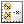Pole-Zero Simplification.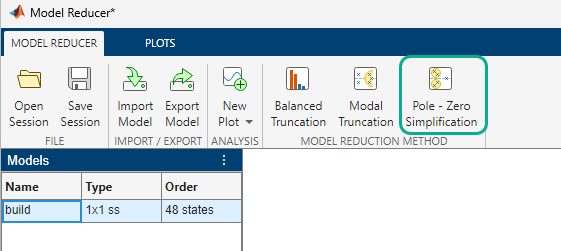In the Pole-Zero Simplification tab, Model Reducer displays a plot of the frequency response of the original model and a reduced version of the model. The app also displays a pole-zero map of both models.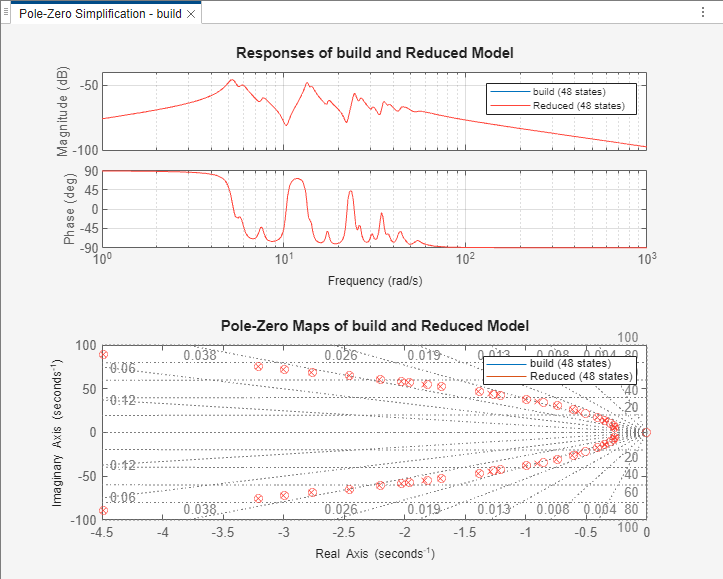The pole-zero map marks pole locations with `x` and zero locations with `o`.

Note

The frequency response is a Bode plot for SISO models, and a singular-value plot for MIMO models.

3. Optionally, change the tolerance with which Model Reducer identifies canceling pole-zero pairs. Model Reducer cancels pole-zero pairs that fall within the tolerance specified by the Simplification of pole-zero pairs value. In this case, no pole-zero pairs are close enough together for Model Reducer to cancel them at the default tolerance of `1e-05`. To cancel pairs that are a little further apart, move the slider to the right or enter a larger value in the text box.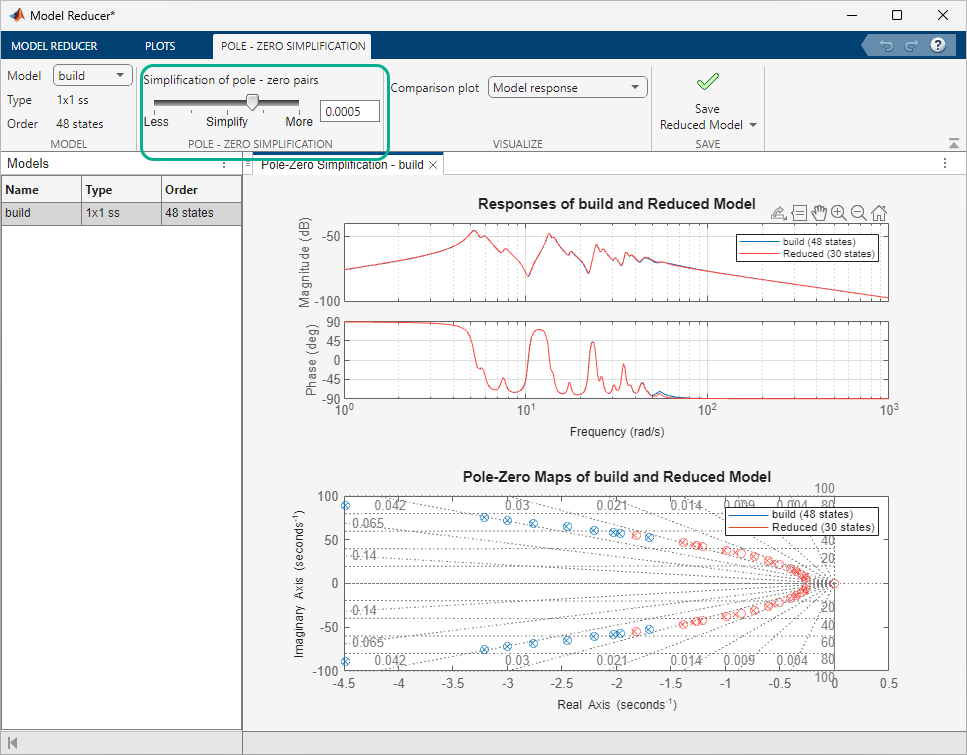The blue `x` and `o` marks on the pole-zero map show the near-canceling pole-zero pairs in the original model that are eliminated from the simplified model. Poles and zeros remaining in the simplified model are marked with red `x` and `o`.

4. Try different simplification tolerances while observing the frequency response of the original and simplified model. Remove as many poles and zeros as you can while preserving the system behavior in the frequency region that is important for your application. Optionally, examine absolute or relative error between the original and simplified model. Select the error-plot type using the buttons on the Pole-Zero Simplification tab.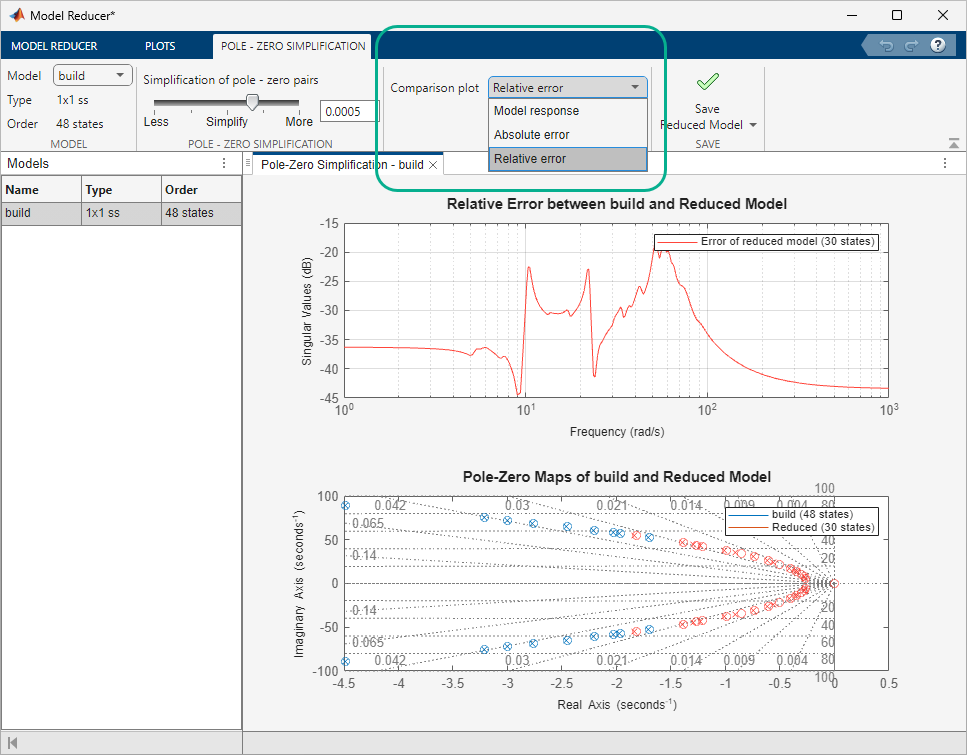For more information about using the analysis plots, see Visualize Reduced-Order Models in the Model Reducer App.

5. When you have a simplified model that you want to store and analyze further, click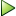. The new model appears in the data browser with a name that reflects the reduced model order.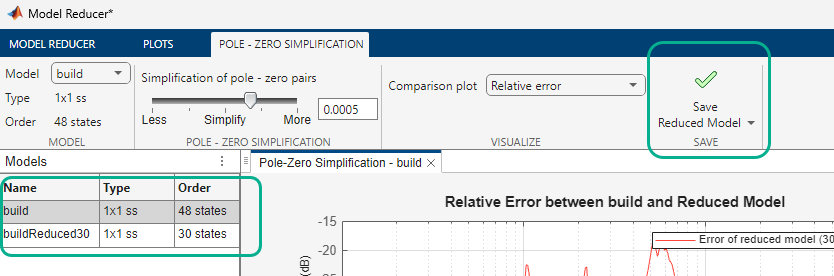After creating a reduced model in the data browser, you can continue changing the simplification parameters and create reduced models with different orders for analysis and comparison.

You can now perform further analysis with the reduced model. For example:

• Examine other responses of the reduced system, such as the step response or Nichols plot. To do so, use the tools on the Plots tab. See Visualize Reduced-Order Models in the Model Reducer App for more information.

• Export reduced models to the MATLAB workspace for further analysis or control design. On the Model Reducer tab, click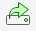Export.

#### Generate MATLAB Code for Pole-Zero Simplification

To create a MATLAB script you can use for further model-reduction tasks at the command line, click Create Reduced Model, and select Generate MATLAB Script.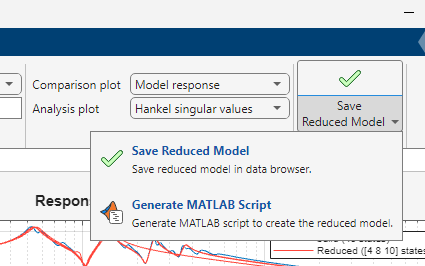Model Reducer creates a script that uses the `minreal` command to perform model reduction with the parameters you have set on the Pole-Zero Simplification tab. The script opens in the MATLAB editor.

### Pole-Zero Cancellation at the Command Line

To reduce the order of a model by pole-zero cancellation at the command line, use `minreal`.

Create a model of the following system, where `C` is a PI controller, and `G` has a zero at $3×1{0}^{-8}$ rad/s. Such a low-frequency zero can arise from derivative action somewhere in the plant dynamics. For example, the plant may include a component that computes speed from position measurements.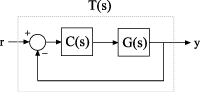```G = zpk(3e-8,[-1,-3],1); C = pid(1,0.3); T = feedback(G*C,1)```
```T = (s+0.3) (s-3e-08) ---------------------- s (s+4.218) (s+0.7824) Continuous-time zero/pole/gain model. ```

In the closed-loop model `T`, the integrator $\left(1/s\right)$ from `C` very nearly cancels the low-frequency zero of `G`.

Force a cancellation of the integrator with the zero near the origin.

`Tred = minreal(T,1e-7)`
```Tred = (s+0.3) (s-3e-08) ---------------------- s (s+4.218) (s+0.7824) Continuous-time zero/pole/gain model. ```

By default, `minreal` reduces transfer function order by canceling exact pole-zero pairs or near pole-zero pairs within `sqrt(eps)`. Specifying `1e-7` as the second input causes `minreal` to eliminate pole-zero pairs within $1{0}^{-7}$ rad/s of each other.

The reduced model `Tred` includes all the dynamics of the original closed-loop model `T`, except for the near-canceling zero-pole pair.

Compare the frequency responses of the original and reduced systems.

```bode(T,Tred,'r--') legend('T','Tred')```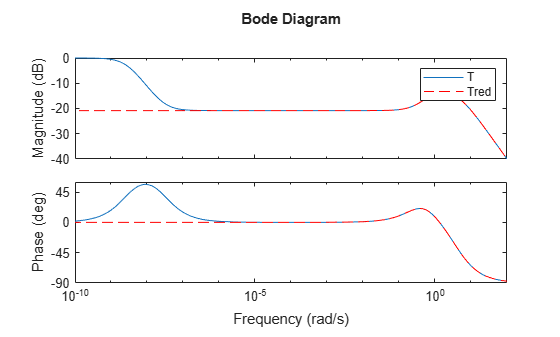Because the canceled pole and zero do not match exactly, some extreme low-frequency dynamics evident in the original model are missing from `Tred`. In many applications, you can neglect such extreme low-frequency dynamics. When you increase the matching tolerance of `minreal`, make sure that you do not eliminate dynamic features that are relevant to your application.